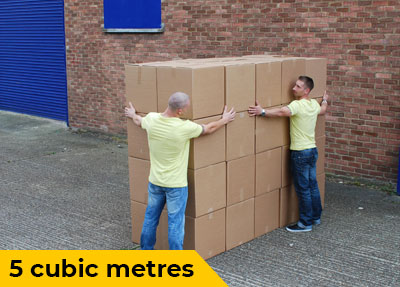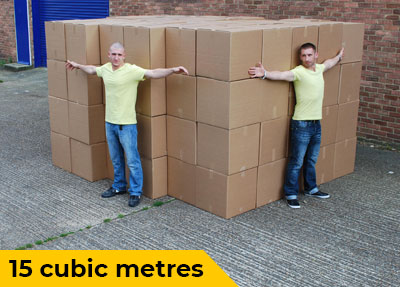VanOne is a trademark of VOGB Ltd, registered and used in the United Kingdom

## Cubic Metre Volume Calculator for Removals

Volume Tool helps you to measure the total volume of items for domestic removal.

Item Name m3 Category
Total 0 m3

Total Items

0

Total m3

0 m3

Total Weight

0 Kg

Total Value

£ 0

## Get an Instant Quote With VanOne

Two men moves are provided by our partner.

Disclaimer: This Volume Tool is designed to estimate the aggregated volume of domestic goods for removals. You can enter the required information to this tool in metres, centimetres and inches but the final cubic calculation result will be shown in cubic metres only.

You don't need to list all the items in one go. You can always come back to your list using the same PC or mobile device (the information is saved in your web browser).

#### Introduction to volume

Look around yourself and you’ll see a lot of living and non-living things of various sizes and shapes. All of these acquire some space. Sometimes it is very useful to measure that space. Measure of space contained or occupied by any form of matter is identified as its volume. Let’s explore this concept further and learn to measure volume of objects accurately. According to Encyclopedia Britannica the most widely used units for measuring volume are cubic metres, cubic centimetres and cubic inches. These units are derived from International System of Units (SI) that serve as a standard and are followed all over the world.

To measure the volume of an object first of all it is necessary to determine whether it has a regular symmetric shape or not. It is easier to measure the volume of symmetric objects like cuboids, prisms and spheres. However measuring the volume of an irregular shaped object involves the effort of splitting it into multiple parts that possess some geometric symmetry and then adding up their volumes to determine the volume of the complete object.

(Diagram 1- 3D images of regular/Irregular objects)

Let’s talk about measuring the volume of a cuboid. Cuboids have six faces and all the opposing sides are parallel to each other. We can determine the Volume (V) of a cuboid by multiplying its length (L), width (W) and height (H).

V = L x W x H

A cube is a cuboid with exactly equal length, width and height. To find the volume of a cube you just have to multiply its length three times.

L = W = H

V = L x L x L

Let’s look at a general form of this formula. We can measure the volume of a cube, cuboid, prism or a cylinder by finding the area of its base and multiplying it with its height.

V = A x H

Some shapes are a little complex as their areas and heights are not geometrically symmetric therefore the formulae for calculating their volumes are also complicated. However these formulae are available everywhere which makes calculating volumes easy for everyone.

Volume of Pyramid or Cone = (A x H)/3

Volume of Sphere = 4/3πr3

All the three dimensional objects have a surface and hence a surface area and volume. The surface only represents the outer layer of an object however the volume takes all the space occupied by that object in account.

A square measure gives us an area however a cubic measure gives us the volume therefore conversion of an area into volume needs that area to be extended along the height. As discussed earlier multiplying the area and height will result in volume with cubic measure.

Diagram 2 (Shapes such as circle, square, rectangle extending along a height)

The number of boxes needed to make one cubic metre depends on the dimensions of the boxes used. As we know that there are hundred centimetres in one meter length. Let’s take one hundred cubic boxes that are simultaneously one centimeter tall, high and deep and place them in a straight line. Then let’s place one hundred straight lines of such boxes side by side to create the base. Now we need to place one hundred boxes of one centimeter cube on each of the boxes that form the base to get a perfect cubic metre. In this way we’ll need 1000000 cubic centimeter boxes to make a cubic metre.

100 x 100 x 100 = 1000000

(Diagram 3)

Let’s try to use fewer boxes to fill up a one cubic centimetre space. Considering there are ten milimetres in a centimetre, let’s use the boxes that are 5 milimetre tall, 5 milimetre wide and 5 milimetre deep. The volume of each of such boxes will be 125 cubic milimetre. We only need four such boxes to fill one cubic centimeter of space.

(Diagram 4)

One inch is approximately equals 25 milimetres. If we use one cubic milimetre boxes to fill up one cubic inch of space we’ll need 25 x 25 x 25 = 15625 boxes.

While converting volume from one unit to another you only need care about the units in consideration and not the object whose volume is being converted into another unit. Whether you are converting the volume of a cube, cylinder, sphere or even an irregular object from one unit to another – just focus on the units and you’ll get the correct conversions.

• From metres to centimetres

To convert cubic metre to cubic centimetre we need to focus on the relation of these two units:

1 m = 100 cm

1 m3 = 100 cm x 100 cm x 100 cm = 1000000 cm3

As one cubic metre is equal to 1000000 cubic centimetres, to convert any volume expressed in cubic metres we just need to multiply it to 1000000 to get it converted in to cubic centimetre.

• From centimetres to metres

For this case we need to divide the volume expressed in cubic centimetres by 1000000 to get it converted in to cubic metres. Let’s convert 500,000 cubic centimetres of volume into cubic metres:

500,000 cm3/ 1000,000 cm3 = 0.5 m3

• From metres to inches

Remember that while converting a unit into a smaller unit you multiply it to scale its value according to the new unit. As one meter is equal to 39.37 inches therefore conversion of cubic metre into cubic inches requires below mentioned multiplication:

V (m3) = V x 39.37 x 39.37 x 39.37 = V (in3)

• From inches to metres

Here we’ll divide the volume in cubic inches three times with 39.37 to make sure that the quantity of volume expressed in cubic metres should remain the same. Numbers will change but the new unit will keep the volume authentic.

V (in3) = V / 39.37 x 39.37 x 39.37 = V (m3)

• From centimetres to inches

This conversion will be based on below mentioned equations:

1 in = 2.54 cm

V (cm3) = V x 2.54 x 2.54 x 2.54 = V (in3)

• From inches to centimetres

V (in3) = V / 2.54 x 2.54 x 2.54 = V (cm3)

Litre is a unit that is mostly used for volume of gases and liquids. One litre is equal to one cubic decimeter therefore converting volume from any other unit requires the conversion of that unit into decimeter and use multiplication or division to ensure the correct conversion.

1 L = 1 dm3

1 dm = 10 cm

1 dm3 = 10 cm x 10 cm x 10 cm = 1000 cm3

Let’s find that how many litres of liquid can be filled in one cubic metre container?

1 m3 = 1000000 cm3

1 dm3 = 1000 cm3

1 m3 = 1000 x 1000 cm3

1 m3 = 1000 dm3

Hence one cubic metre container can accommodate 1000 litres of liquid.

Volume vs mass

Volume and mass are two independent quantities. They are represented by different units. Two objects may have different masses but same volume and vice versa. Two books with same volume and different masses will look similar in size however if you’ll lift them up one of them will be heavier than the other.

Volume vs weight

Weight of a body is equal to its mass multiplied by the gravitational pull. As gravity is a constant we can say that volume and weight are also independent quantities represented by separate units. Have you ever noticed that a litre of honey always weighs more than a litre of water? It is one of the examples that tell us that volume and weight are independent quantities.

Volume vs area

Area of an object has a connection with its volume. Area is a two dimensional quantity that is represented by square metre m2 as its standard unit. Extending any given square metres of area on third axis to any height in metres allows us to calculate the volume in cubic metres using following equation:

V = A x H

Volume vs intensity

You might have heard people talking about volume of workout and intensity of workout however these terms are a little different in scientific context where intensity is measured as amount of pressure per unit area. A nail with a comparatively bigger head is easier to drive into the wall because its big surface area allows it to receive more pressure from the hammer hence the volume of the nail’s head has nothing to do with the intensity, it’s the surface area that matters.

Volume vs pressure

The SI unit of pressure is Pascal (Pa) and according to the standard definition, pressure also depends on surface area and not on the volume however we can imagine that volume of matter can be changed by changing the pressure. This change is very obvious in case of gases and according to Boyle’s law, given the value of pressure and other important factors in SI units, volume can be calculated in cubic metres.

#### How Much Space Does Your Move Need?##### What does 1 cubic metre of moving space look like?##### How much removal van space is 5 cubic metres?##### See what 10 cubic metres look like in a van